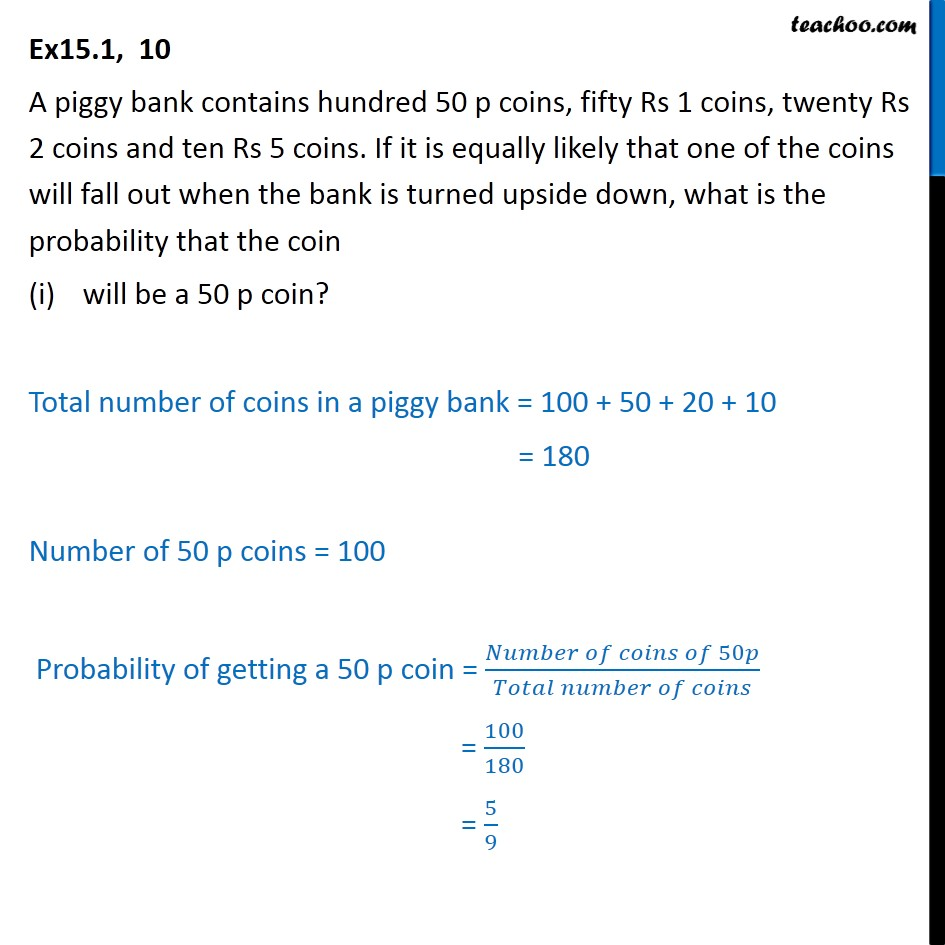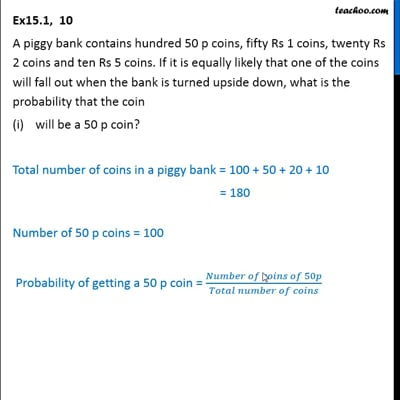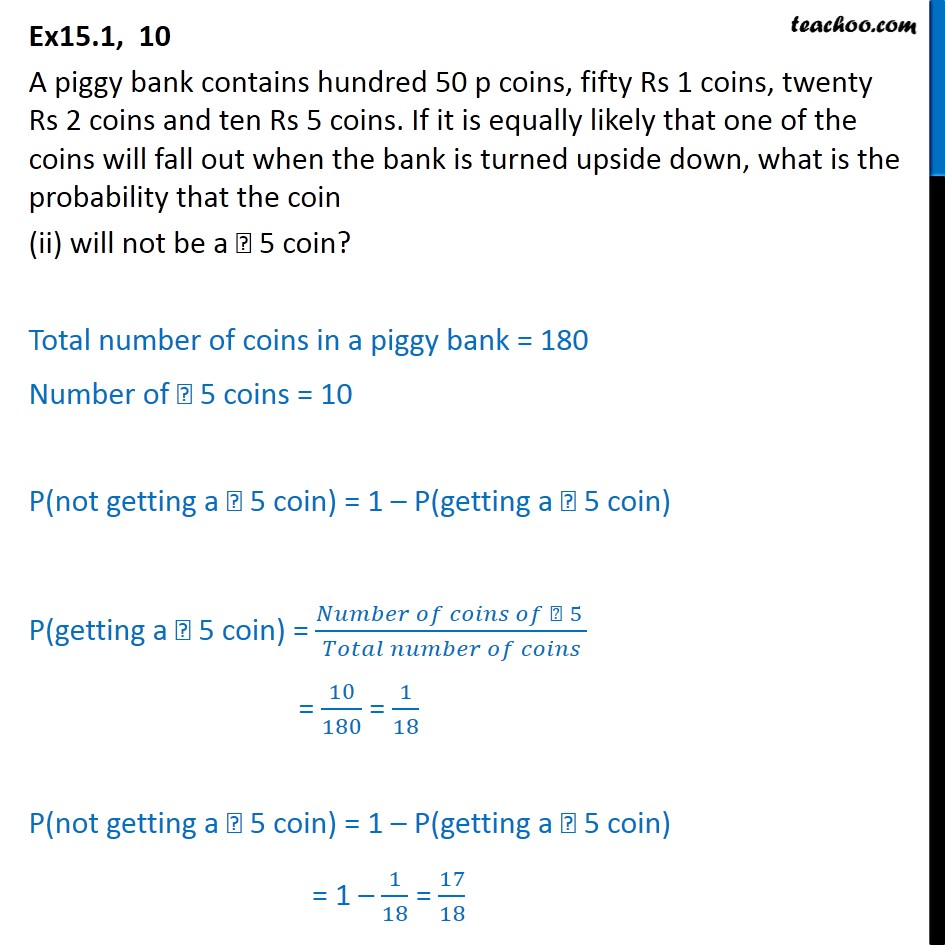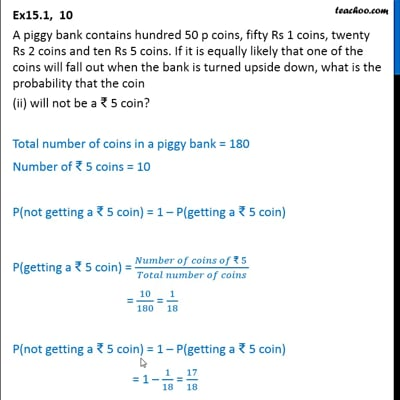Ex 15.1

Chapter 15 Class 10 Probability
Serial order wiseThis video is only available for Teachoo black usersThis video is only available for Teachoo black users

Solve all your doubts with Teachoo Black (new monthly pack available now!)

### Transcript

Ex15.1, 10 A piggy bank contains hundred 50 p coins, fifty Rs 1 coins, twenty Rs 2 coins and ten Rs 5 coins. If it is equally likely that one of the coins will fall out when the bank is turned upside down, what is the probability that the coin will be a 50 p coin? Total number of coins in a piggy bank = 100 + 50 + 20 + 10 = 180 Number of 50 p coins = 100 Probability of getting a 50 p coin = ( 50 )/( ) = 100/180 = 5/9 Ex15.1, 10 A piggy bank contains hundred 50 p coins, fifty Rs 1 coins, twenty Rs 2 coins and ten Rs 5 coins. If it is equally likely that one of the coins will fall out when the bank is turned upside down, what is the probability that the coin (ii) will not be a 5 coin? Total number of coins in a piggy bank = 180 Number of 5 coins = 10 P(not getting a 5 coin) = 1 P(getting a 5 coin) P(getting a 5 coin) = ( 5 )/( ) = 10/180 = 1/18 P(not getting a 5 coin) = 1 P(getting a 5 coin) = 1 1/18 = 17/18